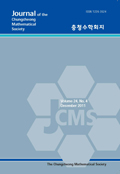상세검색
최근 검색어 전체 삭제
다국어입력
즐겨찾기0KCI등재 학술저널

# A new generalized quartic functional equation and its stability problems

• 등재여부 : KCI등재
• 2020.05
• 205 - 217 (13 pages)
• 2

We will introduce a new type of quartic functional equation and then investigate the stability for a quartic functional equation in a convex modular space.

1. Introduction

2. A solution for a generalized quartic functional equation

3. The Direct Method Approach

4. The Fixed Point Method Approach

References